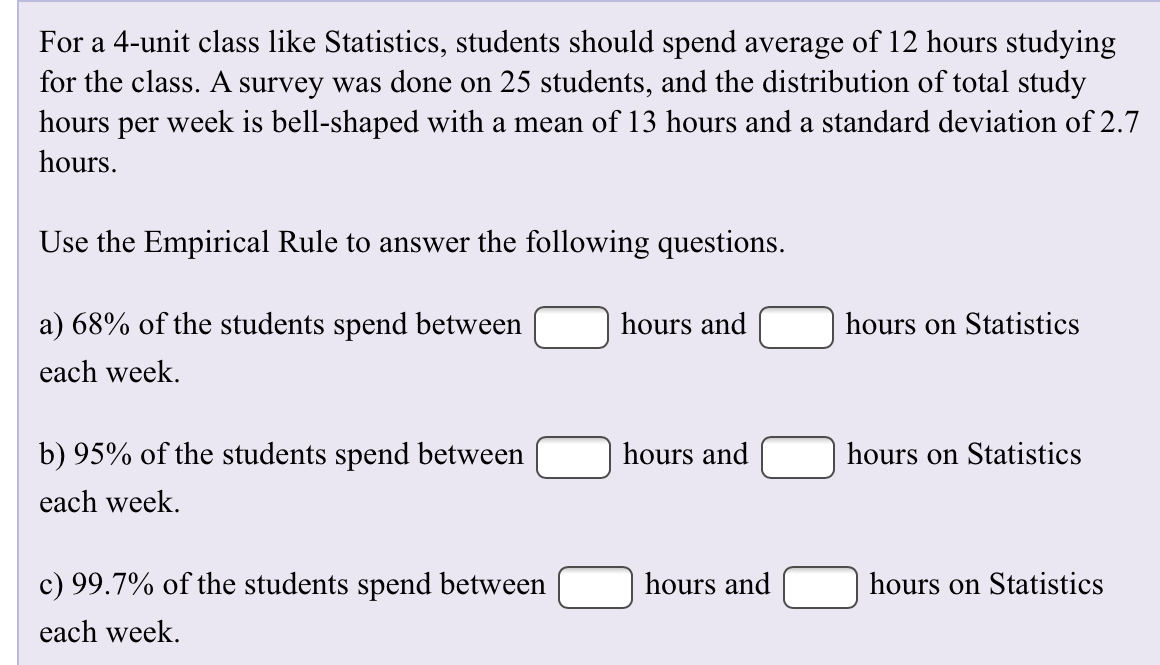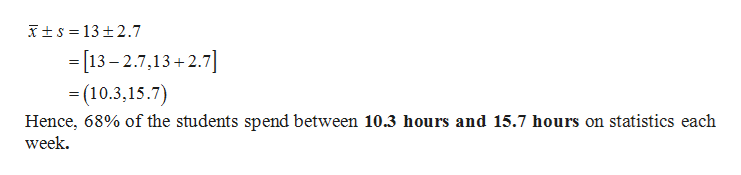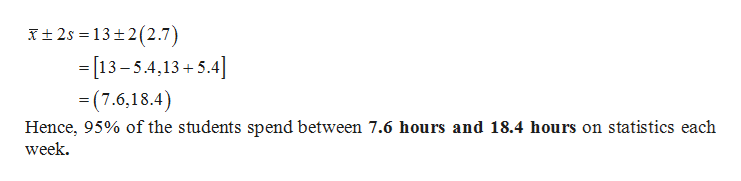# For a 4-unit class like Statistics, students should spend average of 12 hours studyingfor the class. A survey was done on 25 students, and the distribution of total studyhours per week is bell-shaped with a mean of 13 hours and a standard deviation of 2.7hoursUse the Empirical Rule to answer the following questionsa) 68% of the students spend betweenhours andhours on Statisticseach weekhours andb) 95% of the students spend betweenhours on Statisticseach weekc) 99.7% of the students spend betweenhours andhours on Statisticseach week.

Question
257 viewshelp_outlineImage TranscriptioncloseFor a 4-unit class like Statistics, students should spend average of 12 hours studying for the class. A survey was done on 25 students, and the distribution of total study hours per week is bell-shaped with a mean of 13 hours and a standard deviation of 2.7 hours Use the Empirical Rule to answer the following questions a) 68% of the students spend between hours and hours on Statistics each week hours and b) 95% of the students spend between hours on Statistics each week c) 99.7% of the students spend between hours and hours on Statistics each week. fullscreen
check_circle

Step 1

68-95-99.7-rule:

Let X be an observation from a bell shaped distribution, having mean x-bar and sample standard deviation s, then according to the 68-95-99.7-rule, approximately 68%, 95% and 99.7% of the values lie within one, two and three standard deviations of the mean respectively. That is,

Step 2

a.

It is given that the distribution of total study hours per week is bell-shaped with mean 13 hours and standard deviation of 2.7 hours.

It is known that 68% of the data lies within one standard deviations of the mean.help_outlineImage Transcriptionclosets 13 2.7 -[13-2.7,13+2.7] (10.3,15.7) Hence, 68% of the students spend between 10.3 hours and 15.7 hours on statistics each week fullscreen
Step 3

b.

It is known that 95% of the data lies within two standard deviations of the mean. ...help_outlineImage Transcriptioncloset 2s 13 2(2.7) [13-5.4,13+5.4 -(7.6,18.4) Hence, 95% of the students spend between 7.6 hours and 18.4 hours on statistics each week fullscreen

### Want to see the full answer?

See Solution

#### Want to see this answer and more?

Solutions are written by subject experts who are available 24/7. Questions are typically answered within 1 hour.*

See Solution
*Response times may vary by subject and question.
Tagged in
MathStatistics

### Other